Welcome to Wall Street Prep! Use code at checkout for 15% off.Guide to Understanding the Equity Risk Premium (ERP)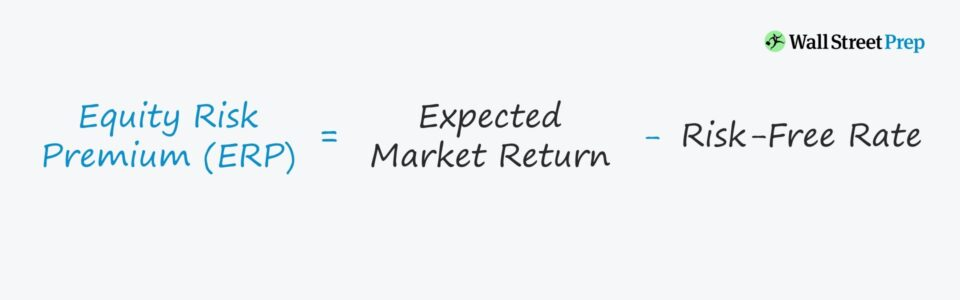## How to Calculate Equity Risk Premium?

The equity risk premium (or the “market risk premium”) is equal to the difference between the rate of return received from riskier equity investments (e.g. S&P 500) and the return of risk-free securities.

The risk-free rate refers to the implied yield on a risk-free investment, with the standard proxy being the 10-year U.S. Treasury note.

The bond issuances by the U.S. government carry “zero risk” since the government could print money if deemed appropriate, so it’d be improbable for the U.S. government to default on its obligations.

No rational investor would accept greater risk in the form of a potential loss of invested capital without the possibility of receiving a higher rate of return – i.e. there must be an economic incentive for investors.

If the potential compensation is inadequate for investors, the risk of owning equities rather than government bonds is not justified.

Unlike a bond with a fixed interest payment schedule and the date of principal repayment, equity securities come with substantially more uncertainty regarding the investment outcome, which is a function of the free cash flow generation and profitability of the underlying company.

The formula for calculating the equity risk premium is as follows.

Equity Risk Premium (ERP) = Expected Market Return (rm)Risk Free Rate (rf)

## Market Risk Premium Calculation Example

Since the projected market return minus the yield on risk-free assets results in the equity risk premium, we can complete a quick calculation example.

Let’s suppose the estimated market return is 8%, while the risk-free rate is 2%. The risk premium is 6% (i.e. 8% – 2%), representing the investor’s expected earnings from the investment in excess of the risk-free rate (rf).

## Market Risk Premium vs. Expected Return: What is the Difference?

In general, a higher equity risk premium corresponds to higher risk in the markets. Therefore, investors should ensure sufficient returns are obtainable from their portfolio of equities.

If the prevailing market valuations remain at the same (or higher) level despite falling equity risk premiums, this could indicate a correction in the stock market could soon occur (i.e. “market bubble”).

Therefore, the equity risk premium tends to increase if the risks and uncertainty in the outlook of the stock market increase (and vice versa).

## What is the Risk Premium Component in CAPM?

The equity risk premium (ERP) is an essential component of the capital asset pricing model (CAPM), which calculates the cost of equity – i.e. the cost of capital and the required rate of return for equity shareholders.

The core concept behind CAPM is to balance the relationship between:

• Capital-at-Risk (i.e. Potential Losses)
• Expected Returns

Here, the proxy for systematic risk (i.e. undiversifiable risk) is the concept of beta, whereas the equity risk premium measures the potential returns, taking into account the risk-free rate.

If plausible, investors seek to obtain the highest potential returns combined with the lowest degree of risk – but the more practical objective is to ensure that the expected returns are reasonable.

The U.S. stock market has averaged a 10-year return of 9.2%, according to research conducted by Goldman Sachs, with a 13.6% annual return in the trailing ten years from 2020 pre-COVID (Source: Capital IQ).

In the same time horizon between 2010 and 2020, the 10-year Treasury note remained in the 2% to 3% range.

There are numerous factors that can impact equity risk premiums, such as:

• Macroeconomic Volatility
• Geopolitical Risks
• Governmental and Political Risk
• Catastrophic Risk and Disasters
• Low Liquidity

## S&P U.S. Equity Risk Premium Index (Historical Chart)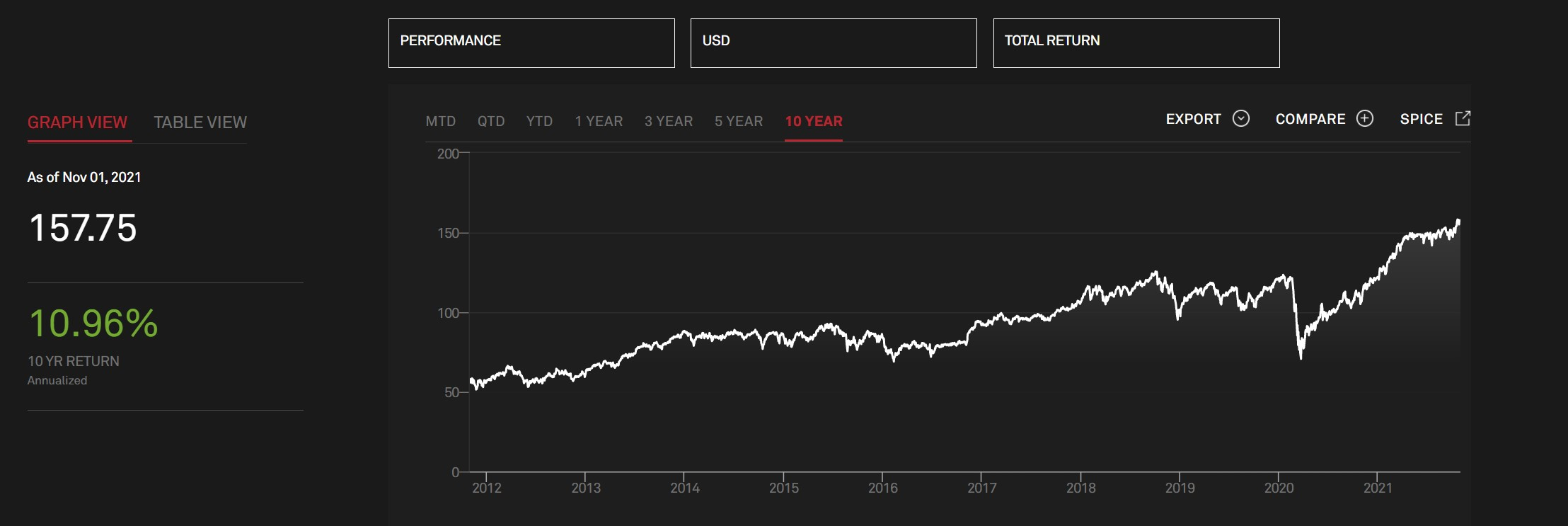10-Year Historical U.S. Equity Risk Premium (Source: S&P Global)

When calculating the cost of equity under the CAPM approach, one common adjustment is called the country risk premium (CRP), which encompasses the same factors as listed in the previous section.

As one would reasonably expect, political instability, economic risks (e.g. recessions, inflation), default risk, and currency fluctuations impact countries unevenly.

For instance, the issue of hyperinflation in Venezuela, which began in 2016, presents a significant country-specific risk that causes instability in all aspects of the country, whether political, socioeconomic, or financial.

With that said, equities in emerging markets come with higher risks, which means higher potential returns to compensate investors.

Cost of Equity = Risk-Free Rate + (Beta× ERP) + Country Risk Premium

Hence, many institutional investment firms nowadays have raised foreign funds to pursue investments outside developed countries.

While part of the reason is diversification, another critical consideration is the limited number of investment opportunities in developed countries that meet their minimum returns hurdle.

Considering that foreign, less developed countries typically have fewer capital providers, outside firms often hold more negotiating leverage – directly leading to more compensation.

We’ll now move to a modeling exercise, which you can access by filling out the form below.Submitting...

## Equity Risk Premium Calculation Example

In the first section of our modeling tutorial, we’ll calculate the equity risk premium.

The two required inputs are listed below:

1. Estimated Market Return
2. Risk-Free Rate

Here, we’ll calculate the ERP for two companies, one based in a developed country while the other is in an emerging market.

Developed Country – Company Assumptions

• Risk-Free Rate (rf) = 2.0%
• Expected Market Return (rm) = 7.5%

Emerging Country – Company Assumptions

• Risk-Free Rate (rf) = 6.5%
• Expected Market Return (rm) = 15%

For both companies, we’ll subtract the risk-free rate from the expected market return to get the following figures for our equity risk premium:

• Developed Market – Company: 5.5%
• Emerging Market – Company: 8.5%

Countries with economies classified as “emerging” markets are less developed economically, so there’s more room for companies to come in and capture market share, but there are also more risks (and required expenditures).

The 5.5% and 8.5% ERP represents the excess return above the risk-free applicable to the appropriate country.

Note that the correct risk-free rate is for the country the company in question does business in, so using the 10-year Treasury note for a company in Japan is incorrect – as a general rule of thumb, the currencies should match.

As affirmed by our example, equity risk premiums tend to be higher in emerging markets than developed markets.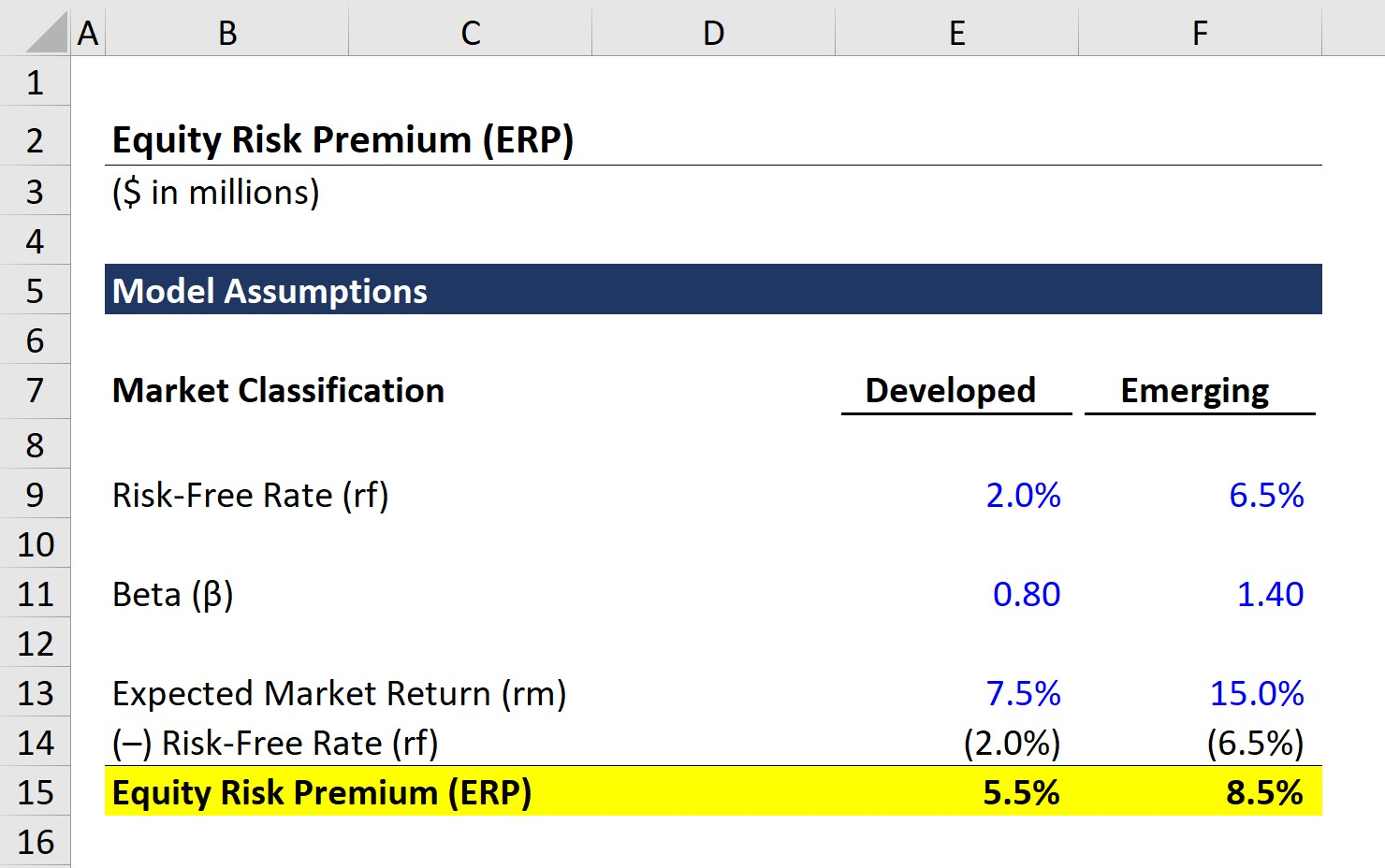## Country Risk Premium and Cost of Equity Calculation

In the next and final part of our modeling exercise, we’ll see how country-specific risks affect the cost of equity calculation under the CAPM approach.

For the company in a developed market (e.g. U.S.), there is no need for a country risk premium (CRP) adjustment.

However, a CRP adjustment would be appropriate for the company in an emerging market (e.g. Argentina, Brazil, Russia).

Here, we’ll assume the 4.0% CRP adjustment is added to the cost of equity calculation, as shown below.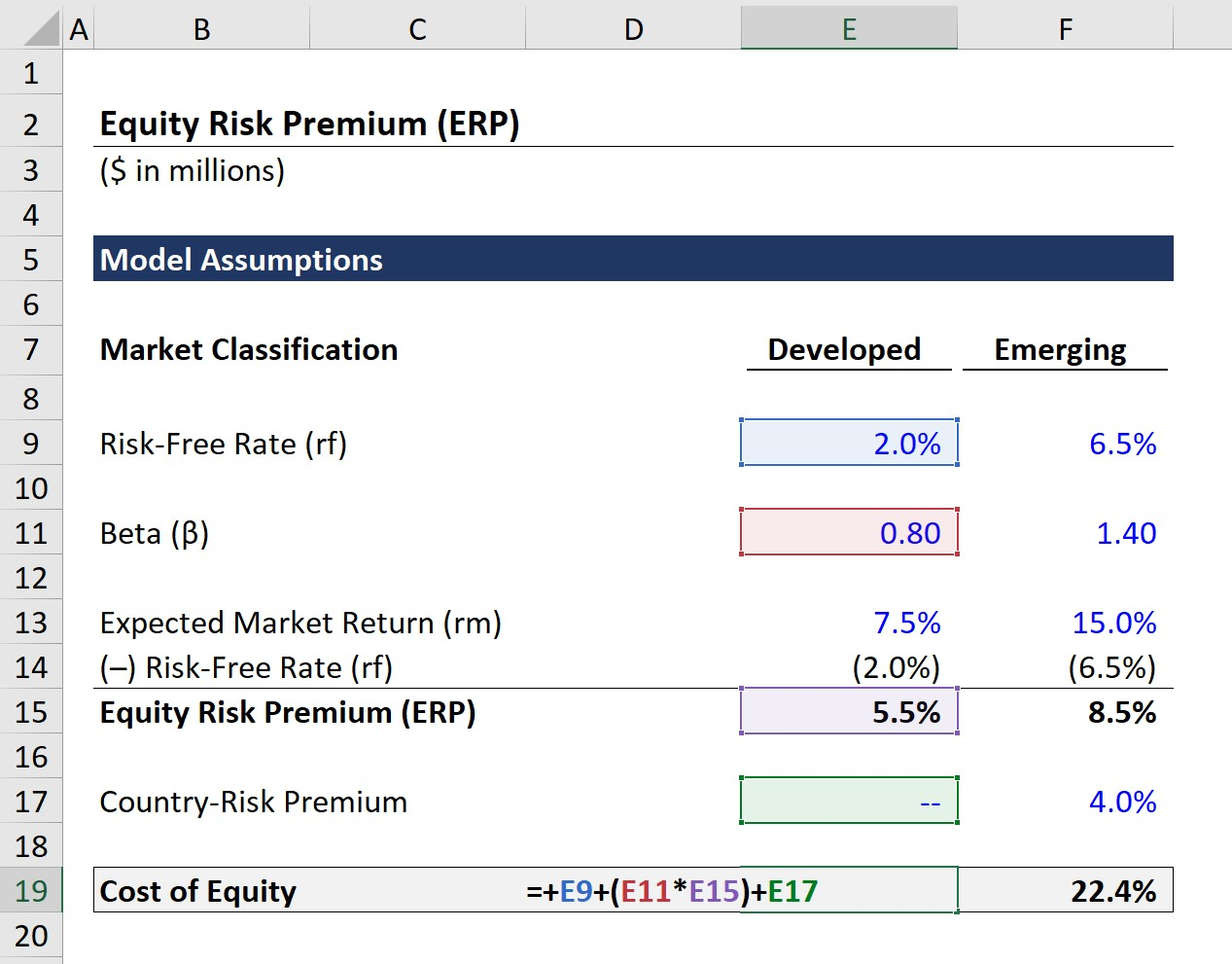From our completed model, the calculated cost of equity is 6.4% and 22.4% in developed and emerging market companies, respectively.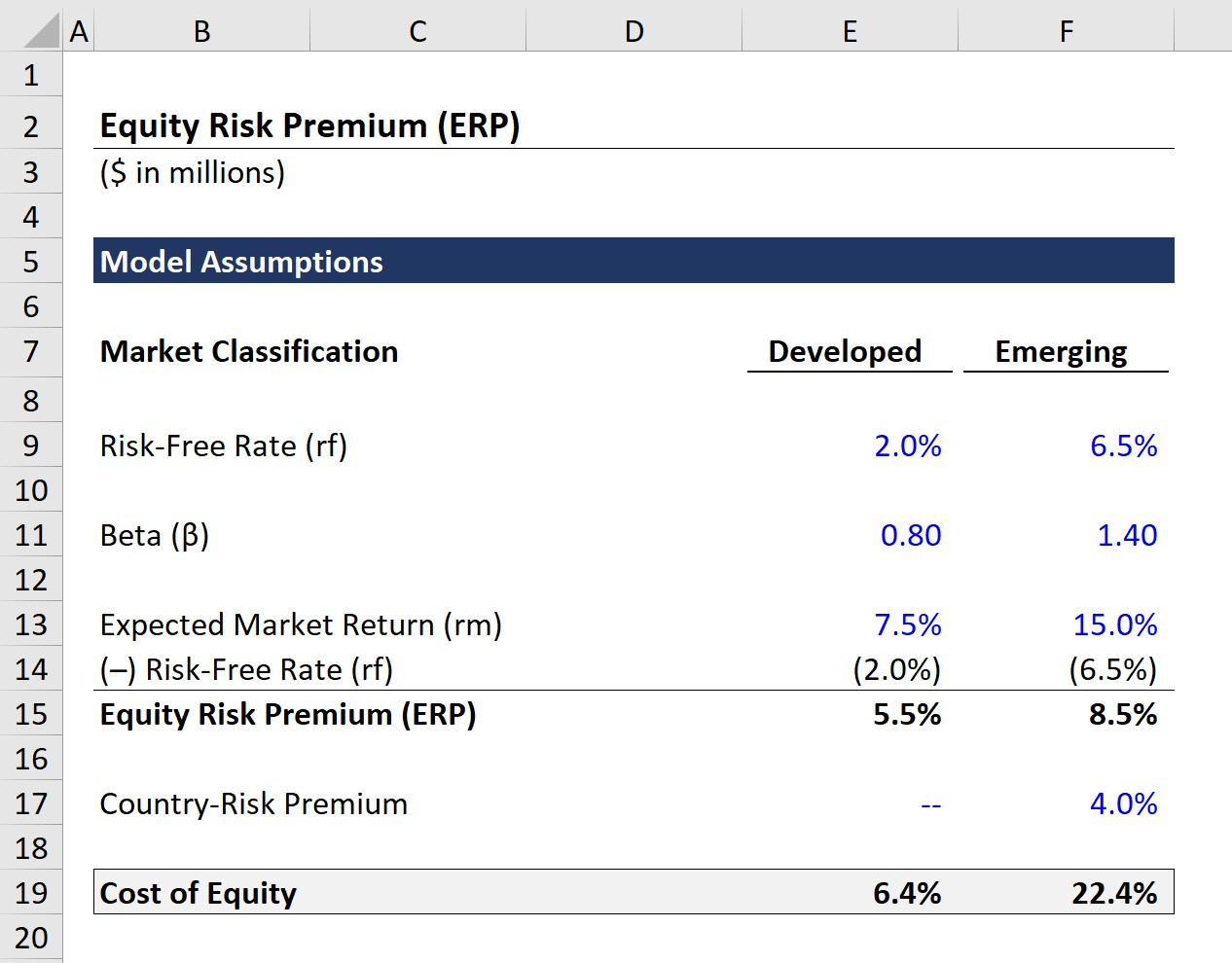Step-by-Step Online Course

### Everything You Need To Master Financial Modeling

Enroll in The Premium Package: Learn Financial Statement Modeling, DCF, M&A, LBO and Comps. The same training program used at top investment banks.

Inline Feedbacks